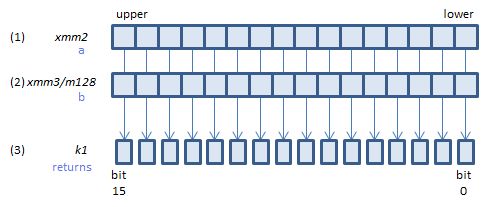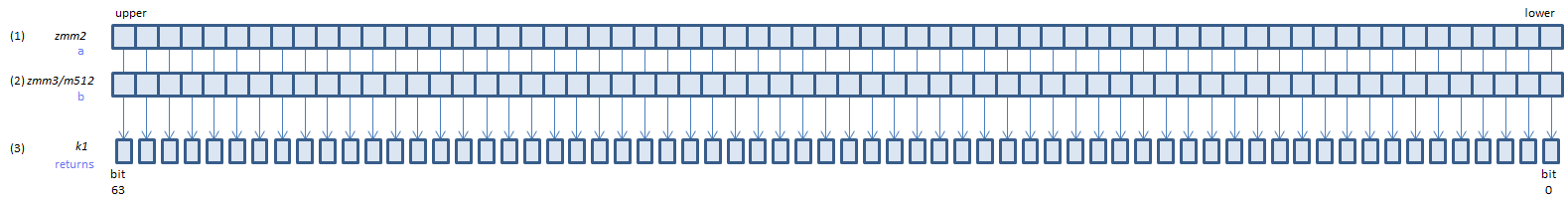﻿ vptestmb

## VPTESTMB - Packed TEST Mask Byte

VPTESTMB k1{k2}, xmm2, xmm3/m128    (V5+BW+VLFor each BYTE, calculate bitwise (1) & (2), if any bit of the result is 1, set 1, else set 0, to the corresponding bit of (3).
If k2 bit is 0, the calculation is not done and the corresponding bit of (3) is set to zero. Upper bits of (3) are zero cleared.
VPTESTMB k1{k2}, ymm2, ymm3/m256    (V5+BW+VL